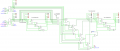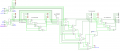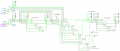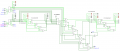# Logisim simulation of subtraction using 2's complement: When to apply 2's complement to output?

Joined Jul 6, 2021
6
Hi,
For the benefit of students (and in this case I now have to include myself as one too) I am simulating the use of binary addition to carry out a subtraction using 2's complement. I have no issue with applying 2's complement to the subtrahend, and adding it to the minuend. For example 3-2 = 1, where I first take the 2's complement of 2 and then add it to 3). It is my understanding (and my Logisim model plays true to this) that I then apply 2's complement to the output of this addition to produce the final answer, if no carry occurs. If a carry does occur, just drop it and ignore the final step of applying 2's complement, as you already have the answer.

All this sounds simple enough, and it works in every instance, EXCEPT where I subtract zero! If I attempt to subtract zero, the hard logic says I should apply 2's complement, when clearly I should not. I have also attempted to resolve this by monitoring for overflow (which is subtly different to a carry over) but again the logic does not stack up. I have looked everywhere online and can find no solution for how the discrete logic should look to determine whether or not to apply that final 2's complement. I understand I could apply an array of logic gates (OR gates for example) to determine whether or not the subtrahend = 0, however that seems over the top. Funnily enough nobody speaks of this complete anomaly online, that subtracting zero essentially subverts the process of applying 2's complement for the final answer.

Here are some images (sorry they are a bit small to encompass the entire project for the sake of clarity) to demonstrate. Note the carry flag LED is set appropriately for all options, except where I subtract zero, in which case (if I applied the glue logic here) it would insist on applying 2s complement which is wrong!Correct! 3-2 = 1Correct! 3-3 = 0Correct! 3-4 = -1 (applying 2s compliment as per carry bit)Incorrect! 3-0 = -13 (applying 2s compliment as per carry bit)

NOTE: I am well aware the implementation of this (2's complement subtraction using addtion) logic could be done in a smarter and more integrated manner, however for teaching purposes I am attempting to use glue logic here in as discrete a fashion as possible to better convey the underlying principles.

#### Attachments

• 3.6 KB Views: 35

Joined Jul 6, 2021
6
I have also included the Logisim project file above, but neglected to include 2 library components that you will need in order to run it. Here they are

#### Attachments

• 3.3 KB Views: 22

#### Papabravo

Joined Feb 24, 2006
20,378
I don't think you need to apply the 2's complement twice. To do a subtraction you add the 2's complement of the subtrahend and you are done! Period. Full Stop.

In a 1's complement adder you have to do an end around carry. Maybe that is what you are thinking of.

BTW - the 2's complement of 0 is ....ta-da 0!

So subtracting zero and adding zero gives you back the original value of the other operand.

•click_here and ZCochran98

#### ZCochran98

Joined Jul 24, 2018
273
I don't think you need to apply the 2's complement twice. To do a subtraction you add the 2's complement of the subtrahend and you are done! Period. Full Stop.

In a 1's complement adder you have to do an end around carry. Maybe that is what you are thinking of.

BTW - the 2's complement of 0 is ....ta-da 0!

So subtracting zero and adding zero gives you back the original value of the other operand.
You beat me to it by about half a second.
Basically, your circuitry is working correctly, except for the second 2's compliment, which is unnecessary.

#### Papabravo

Joined Feb 24, 2006
20,378
You beat me to it by about half a second.
Basically, your circuitry is working correctly, except for the second 2's compliment, which is unnecessary.
Great minds think alike, and simultaneously to boot.

•ZCochran98

Joined Jul 6, 2021
6
I don't think you need to apply the 2's complement twice. To do a subtraction you add the 2's complement of the subtrahend and you are done! Period. Full Stop.

In a 1's complement adder you have to do an end around carry. Maybe that is what you are thinking of.

BTW - the 2's complement of 0 is ....ta-da 0!

So subtracting zero and adding zero gives you back the original value of the other operand.
Yes, it is certainly very neat how when applying 2's complement to 0, you still end up with 0. It avoids 0 having 2 representations in the numbering system (eg -0, which makes no sense). If you look at my images though, you can see that, where the carry is set, the final answer does not require the application of a second 2's complement at the output. However where the carry is clear, you do. People are welcome to download my Logisim example too, and have a play and you will see exactly what i mean. I have used the little red LED's in the schematic to make it easier to understand the logic.

•jjw

Joined Jul 6, 2021
6
I realize that several people here are saying the same thing, that a second 2's complement being applied to the output is not necessary, and that's more or less what I have thought half my adult life. However now that I have the logic laid out, everything appears correct and working, and yet... 3 - 4 = 15 (or -7 if you assume the MSb to be a sign bit, but either way it's not correct). Something is wrong, either in my Logisim setup, my logic, or the universe (and I'm betting it aint the universe)

Joined Jul 6, 2021
6
I realize that several people here are saying the same thing, that a second 2's complement being applied to the output is not necessary, and that's more or less what I have thought half my adult life. However now that I have the logic laid out, everything appears correct and working, and yet... 3 - 4 = 15 (or -7 if you assume the MSb to be a sign bit, but either way it's not correct). Something is wrong, either in my Logisim setup, my logic, or the universe (and I'm betting it aint the universe)
Sorry, I obviously wrote that late at night and am confusing myself at this point! Wish there was a way to edit/delete something after the fact. Anyway, my problem is that my carry logic works as prescribed by other websites, where a clear carry flag means you need to apply a 2's complement to the output, and a set carry flag means you already have the final answer from the 2's complement subtraction (and therefore do not need to apply 2's complement to the output), however this logic does not hold true when subtracting 0. IE 5 - 0 = 5 (with carry flag clear, but this means I should apply 2's complement to the (already correct) output for the final answer, which is INCORRECT!)

As long as my subtrahend <> 0, the logic around the carry flag determining whether or not to apply the output 2's complement is correct. If subtrahend = 0 the wheels fall off with this logic.

Would really appreciate if somebody could clarify how the logic should work around this peculiarity, thank you.

#### click_here

Joined Sep 22, 2020
548

Think of an analogue clock - There are 2 ways of going from 2pm to 1pm:
1) you subtract 1h

2's/C is like the second type

Looking at a 4bit number, 0's 2's/C is...
input = 0000
step1 = ~input = 1111
step2 = step1 + 1 = 0000

i.e.
0 = -0

#### BobTPH

Joined Jun 5, 2013
7,517
A twos complement subtract is done simply by complementing (logical NOT) the second operand and adding it to the first with a forced carry.

The forced carry turns the simple complement into a twos complement.

Bob

Joined Jul 6, 2021
6

Think of an analogue clock - There are 2 ways of going from 2pm to 1pm:
1) you subtract 1h

2's/C is like the second type

Looking at a 4bit number, 0's 2's/C is...
input = 0000
step1 = ~input = 1111
step2 = step1 + 1 = 0000

i.e.
0 = -0
Yep, I definitely understand that. What I am saying is that my logic works just fine as long as I am subtracting anything other than 0. If I try to subtract zero, the carry flag logic is wrong, and I am wondering how digital electronic designers deal with this problem. The rude/crude method would be for me to use some additional logic to detect when the subtrahend is 0, and then override the logics "urge" to apply the 2's complement at the output which introduces the error. this really seems wasteful of additional gates, and I am wondering what people normally do to overcome this problem.

If you look at my original question (images) you will see what I mean.

#### Deleted member 115935

Joined Dec 31, 1969
0
2's compliment is a number system,

if you represent A and B as 2's compliment, and add or subtract them,
then the answer is 2's compliment.

Now as you have seen,
to convert a +ve number in 2's compliment to straight binary, then you just drop the top bit.

to convert a negative number to straight binary
One way is to invert all the bits and add 1.

but as far as the original question is concerned,

if you put in two 2's compliment numbers, then you get out 2's compliment,.

If you put in A as 2's compliment, and B as streight binary,
then the output is "unknown" format
unless, as we have seen, the B is a positive number, in which case the 2's compliment and the straight binary representation are the same.

#### click_here

Joined Sep 22, 2020
548
Have a look online at how a 2's/c is usually done with logic gates.